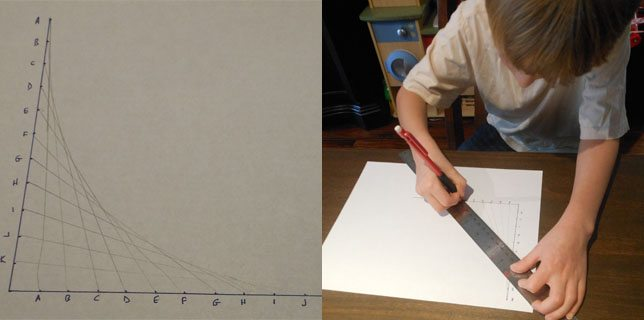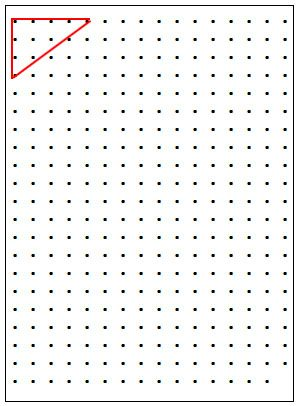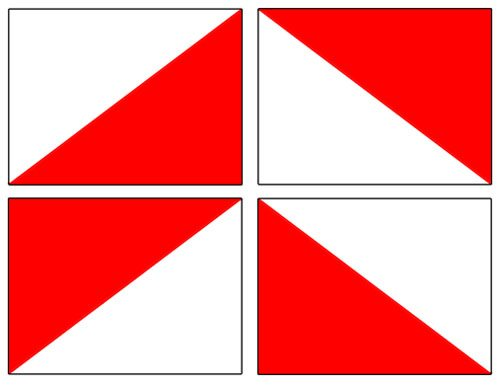# GeekDad Puzzle of the Week Solution – Wacky Right Integer String Triangles

Last week’s puzzle, dutifully recreated for your benefit:

This weekend, Max and I were playing at making curves out of all straight lines. After showing him a simple Bézier curve on paper, we decided to do it with string. We created a grid of nails on a plank of wood, and the matrix included a total of 335 nails. (The grid started out as 21 x 16, but the lower right nail kept falling out, so we removed it.) It looked something a little like this:Max took out some string and after a few moments, made a 3-4-5 right triangle in the corner. He noted that the length of string it took was a whole number (12), and stated that that was the only right triangle he could make with a whole number length of string.I quickly made a second 3-4-5 right triangle elsewhere in the grid, and at that moment, this week’s GeekDad Puzzle of the Week dawned on me: Just how many distinct right triangles (of any size, shape, or orientation) could we make on this (almost) rectangular grid with integer lengths of string?

For purposes of this puzzle, assume that the nails are simply points (no width) and that no straight lines between nails needed are diverted by intermediate / uninvolved nails.

There were basically two types of responses we saw to this week’s puzzle: “brute force” and “mathematical elegance.”

The “brute force” methods simply wrote a straightforward program to cycle through each combination of three nails in the grid, determine the distances between them, and if they both made a right triangle and were integer in length, they added to the count. To this end, depending upon initial assumptions coded in, there were some 335 x 334 x 333 = 37,259,370 different triangles that could be tested. The execution time took on the order of 30 seconds or so, once again varying upon the language and how tightly the code was written.

The advantage of the “brute force” is that it tests each and every possible combination, and would catch any oddly rotated right triangles; that is, it would catch any triangles that don’t have their non-hypotenuse sides perpendicular to the axes. The disadvantage, of course, is that you had to code (not everyone has dev skills.)

The “mathematical elegance” was more approachable for those that remembered at least basic geometry and a little combinatorics. A quick web search or some time in your favorite spreadsheet program could identify all of the integer right triangles that could fit at least once in a 15 x 20 grid. (Assume that the nail that kept falling out is in place; we can account for it later!) If we defined a bounding rectangle around each in each orientation, we could see how many distinct places the bounding rectangles could fit in the 15 x 20 grid and then do some multiplication and addition to reach the answer.

Let’s start with the 3-4-5 right triangle that Max drew. One bounding rectangle would be 4 x 3, and in the diagram below, we can see how many times the bounding rectangle would fit in a completed 15 x 20 grid:Here, we can see that the 4 x 3 grid can fit in 12 different positions from left to right, and 18 from top to bottom. More generally, and m x n box can fit in an p x q grid some (p + 1 – m) times horizontally, and (q + 1 – n) times vertically as (15 + 1 – 4) = 12 and (20 + 1 – 3) = 18. This means that the 4 x 3 rectangle can be place in 12 x 18 = 216 distinct locations. If we rotate the rectangle to be 3 x 4, it will fit (15 + 1 – 3) = 13 times horizontally, and (20 + 1 – 4) = 17 times vertically, and 13 x 17 = 221 distinct locations. Adding 216 + 221 = 437 distinct locations for a rectangle that is 3 units on one side and 4 on the other.

Within that 4 x 3, there are four different orientations in which the 3-4-5 triangle can be drawn: lower right, upper right, lower left, and upper left as shown below:Therefore, for a 3-4-5 right triangle, there are 437 x 4 = 1,748 different ways it could be placed in a full 15 x 20 grid of 16 nails across and 21 pins down.

More generally, in a full p x q grid, an integer right triangle orthogonal to the axes with sides m and n can be placed distinctly the following number of ways:

4 x [(p + 1 – m) x (q + 1 – n) + (p + 1 – n) x (q + 1 – m)]

For each triangle size with any freedom within the 15 x 20 complete grid, we get:

Triangle Size Distinct Placements in full 15 x 20 grid
3-4-5 triangle 1748
5-12-13 triangle 652
6-8-10 triangle 1000
8-15-17 triangle 244
9-12-15 triangle 444
12-16-20 triangle 80

Of course, for the 15-20-25 triangle, there are only four distinct placements in the full grid, as each would take up the full grid. That is, there is only only position for the bounding rectangle, and four rotations as shown above.

So, what about the nail that kept falling out?

Only 4 distinct placements of each size completely occupy the lower right corner where the void / missing nail resides. Of those, only three actually use that nail; the upper left rotation wouldn’t use that nail spot. If we subtract 3 from each of the distinct placement counts possible in a full 15 x 20 grid (above), we have our answer.

There are a total of 1748 – 3 + 652 – 3 + 1000 – 3 + 244 – 3 + 444 – 3 + 80 – 3 + 4 – 3 = 4,136 distinct right triangles that can be created with whole number lengths of string on our board. This matches exactly the solution from my 50 some-odd lines of interpreted code I hacked together to get a quick answer.

Wheh!

Congratulations to V. Washington for submitting a correct answer and for being drawn out of all of the correct answers submitted. This week’s \$50 ThinkGeek Gift Certificate is his/hers!

Thanks to everyone that sent in a response. Happy puzzling!